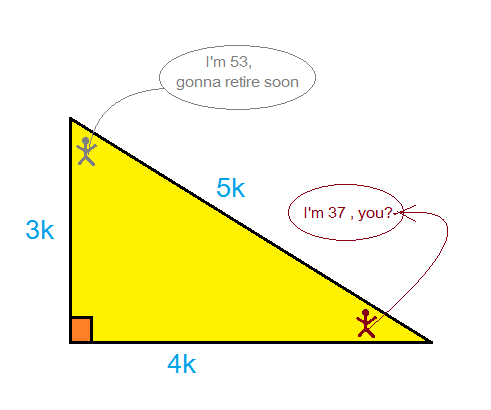# Simply funny or Funnily simple?

Geometry Level 2Given that the triangle which has sides in the ratio $3:4:5$, has 2 angles approximately equal to $37^\circ$ and $53^\circ$, which of the following values can be found by using simple trigonometric identities?

×